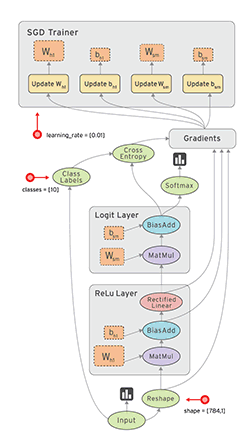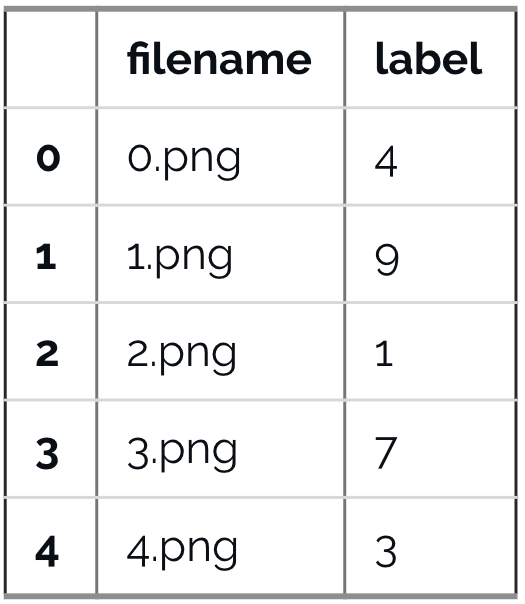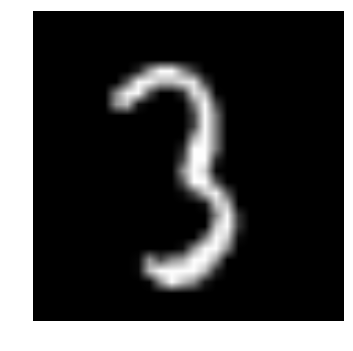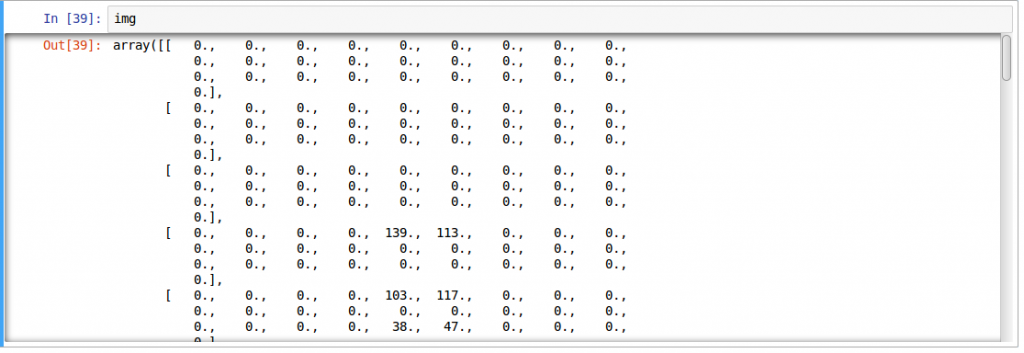{{announcement.body}}
{{announcement.title}}

# An Introduction to Implementing Neural Networks Using TensorFlow

DZone 's Guide to

# An Introduction to Implementing Neural Networks Using TensorFlow

· AI Zone ·
Free Resource

Comment (0)

Save
{{ articles.views | formatCount}} Views

If you have been following the world of data science/machine learning, you just can’t miss the buzz around deep learning and neural networks. Organizations are looking for people with deep learning skills wherever they can use them. From running competitions to open-sourcing projects and paying big bonuses, people are trying every possible thing to tap into this limited pool of talent.

If you are excited by the prospects deep learning has to offer but have not started your journey yet, I am here to enable it.

Let’s get started!

## When to Use Neural Networks

Neural networks have been in the spotlight for quite some time now. For a more detailed explanation on neural network and deep learning, see here. Its “deeper” versions are making tremendous breakthroughs in many fields such as image recognition, speech and natural language processing, etc.

The main question that arises is when to and when not to apply neural networks. This field is like a gold mine right now, with many discoveries uncovered every day. And to be a part of this “gold rush,” you have to keep a few things in mind:

• Firstly, neural networks require clear and informative data (and mostly big data) to train. Try to imagine neural networks as a child. It first observes how its parent walks. Then it tries to walk on its own, and with its every step, the child learns how to perform a particular task. It may fall a few times, but after few unsuccessful attempts, it learns how to walk. If you don’t let it walk, it might never learn how to walk. The more exposure you can provide to the child, the better it is.
• It is prudent to use neural networks for complex problems such as image processing. Neural nets belong to a class of algorithms called representation learning algorithms. These algorithms break down complex problems into simpler form so that they become understandable (or “representable”). Think of it as chewing food before you gulp. This would be harder for traditional (non-representation learning) algorithms.
• When you have the appropriate type of neural network to solve the problem. Each problem has its own twists. The data decides the way you solve the problem. For example, if the problem is of sequence generation, recurrent neural networks are more suitable, whereas if it is an image-related problem, you would probably be better of taking convolutional neural networks for a change.
• Last but not the least, hardware requirements are essential for running a deep neural network model. Neural nets were “discovered” long ago, but they have been shining in recent years for the main reason that computational resources are better and more powerful. If you want to solve a real-life problem with these networks, get ready to buy some high-end hardware!

## How to Solve Problems With Neural Networks

Neural networks are a special type of machine learning (ML) algorithm. So as with every ML algorithm, it follows the usual ML workflow of data preprocessing, model building, and model evaluation. For the sake of conciseness, I have listed out a to-do list of how to approach a neural network problem.

• Check whether it is a problem where neural networks give you uplift over traditional algorithms (refer to the checklist in the section above).
• Do a survey of which neural network architecture is most suitable for the required problem.
• Define neural network architecture through whichever language/library you choose.
• Convert data to right format and divide it into batches.
• Pre-process the data according to your needs.
• Augment data to increase size and make better-trained models.
• Feed batches to a neural network.
• Train and monitor changes in training and validation datasets.
• Test your model, and save it for future use.

For this article, I will be focusing on image data. Let's understand that first before we delve into TensorFlow.

## Understanding Image Data and Popular Libraries to Solve It

Images are mostly arranged as 3D arrays, with the dimensions referring to height, width, and color channel. For example, if you take a screenshot of your PC at this moment, it would be first converted into a 3D array and then compress it PNG or JPG file formats.

While these images are pretty easy to understand to a human, a computer has a hard time understanding them. This phenomenon is called a semantic gap. Our brain can look at the image and understand the complete picture in a few seconds. On the other hand, the computer sees an image as just an array of numbers. So the problem is, how do we explain this image to the machine?

In early days, people tried to break down the image into “understandable” format for the machine like a “template.” For example, a face always has a specific structure that is somewhat preserved in every human, such as the position of our eyes and nose, or the shape of our face. But this method would be tedious because when the number of objects to recognize would increase, the “templates” would not hold.

Fast forward to 2012, and a deep neural network architecture won the ImageNet challenge, a prestigious challenge to recognize objects from natural scenes. It continued to reign its sovereignty in all the upcoming ImageNet challenges, thus proving the usefulness to solve image problems.

So which library/language do people normally use to solve image recognition problems? One recent survey I did showed that most of the popular deep learning libraries have an interface for Python, followed by Lua, Java, and Matlab. The most popular libraries, to name a few, are:

Now, that you understand how an image is stored and which are the common libraries used, let us look at what TensorFlow has to offer.

## What Is TensorFlow?

“TensorFlow is an open source software library for numerical computation using dataflow graphs. Nodes in the graph represents mathematical operations, while graph edges represent multi-dimensional data arrays (aka tensors) communicated between them. The flexible architecture allows you to deploy computation to one or more CPUs or GPUs in a desktop, server, or mobile device with a single API.”If that sounds a bit scary, don’t worry. Here is my simple definition. Look at TensorFlow as nothing but numpy with a twist. If you have worked on numpy before, understanding TensorFlow will be a piece of cake! A major difference between numpy and TensorFlow is that TensorFlow follows a lazy programming paradigm. It first builds a graph of all the operations to be done, and then when a “session” is called, it “runs” the graph. It’s built to be scalable, by changing internal data representation to tensors (AKA multi-dimensional arrays). Building a computational graph can be considered as the main ingredient of TensorFlow. To learn more about mathematical constitutions of a computational graph, read this article.

It’s easy to classify TensorFlow as a neural network library, but it’s not just that. Yes, it was designed to be a powerful neural network library. But it has the power to do much more than that. You can build other machine learning algorithms on it such as decision trees or k-nearest neighbors. You can literally do everything you normally would do in numpy! It’s aptly called “numpy on steroids.”

The advantages of using TensorFlow are:

• It has an intuitive construct because, as the name suggests, it has a “flow of tensors.” You can easily visualize each and every part of the graph.
• Easily train on CPU/GPU for distributed computing.
• Platform flexibility. You can run the models wherever you want, whether it is on mobile, server or PC.

## A Typical “Flow” of TensorFlow

Every library has its own “implementation details,” i.e. a way to write that follows its coding paradigm. For example, when implementing scikit-learn, you first create an object of the desired algorithm, then build a model on the training set and get predictions on the testing set — something like this:

``````# define hyperparamters of ML algorithm
clf = svm.SVC(gamma=0.001, C=100.)
# train
clf.fit(X, y)
# test
clf.predict(X_test)``````

As I said earlier, TensorFlow follows a lazy approach. The usual workflow of running a program in TensorFlow is as follows:

• Build a computational graph. This can be any mathematical operation TensorFlow supports.
• Initialize variables to compile the variables defined previously
• Create session this is where the magic starts!
• Run graph in session the compiled graph is passed to the session, which starts its execution.
• Close session shutdown the session.

A few terminologies used in TensorFlow:

``placeholder: A way to feed data into the graphs``
``feed_dict: A dictionary to pass numeric values to computational graph``

Let's write a small program to add two numbers!

``````# import tensorflow
import tensorflow as tf

# build computational graph
a = tf.placeholder(tf.int16)
b = tf.placeholder(tf.int16)

# initialize variables
init = tf.initialize_all_variables()

# create session and run the graph
with tf.Session() as sess:
sess.run(init)

# close session
sess.close()``````

## Implementing Neural Networks in TensorFlow

Note: We could have used a different neural network architecture to solve this problem, but for the sake of simplicity, we settle on feedforward multilayer perceptron with an in-depth implementation.

Let's remember what we learned about neural networks first.

A typical implementation of neural network would be as follows:

• Define the neural network architecture to be compiled
• Transfer data to your model
• Under the hood, the data is first divided into batches so that it can be ingested. The batches are first preprocessed, augmented and then fed into neural networks for training.
• The model then gets trained incrementally.
• Display the accuracy for a specific number of timesteps.
• After training save the model for future use.
• Test the model on a new data and check how it performs.

Here, we solve our deep learning practice problem for identifying the digits. Let’s for a moment take a look at our problem statement.

Our problem with image recognition to identify digits from a given 28x28 image. We have a subset of images for training and the rest for testing our model. So first, download the train and test files. The dataset contains a zipped file of all the images in the dataset and both the `train.csv` and `test.csv` have the name of the corresponding train and test images. Any additional features are not provided in the datasets, just the raw images are provided in ‘.png’ format.

As you know we will use TensorFlow to make a neural network model. So you should first install TensorFlow in your system. Refer to the official installation guide for installation, as per your system specifications.

We will follow the template as described above. Create a Jupyter notebook with Python 2.7 kernel and follow the steps below.

Import all the required modules:

``````%pylab inline

import os
import numpy as np
import pandas as pd
from sklearn.metrics import accuracy_score
import tensorflow as tf``````

Set a seed value so that we can control our model's randomness:

``````# To stop potential randomness
seed = 128
rng = np.random.RandomState(seed)``````

The first step is to set directory paths for safekeeping!

``````root_dir = os.path.abspath('../..')
data_dir = os.path.join(root_dir, 'data')
sub_dir = os.path.join(root_dir, 'sub')

# check for existence
os.path.exists(root_dir)
os.path.exists(data_dir)
os.path.exists(sub_dir)``````

Let's read our datasets. These are in CSV format and have a filename along with the appropriate labels:

``````train = pd.read_csv(os.path.join(data_dir, 'Train', 'train.csv'))Let's see what our data looks like! We read our image and display it.

``````img_name = rng.choice(train.filename)
filepath = os.path.join(data_dir, 'Train', 'Images', 'train', img_name)

pylab.imshow(img, cmap='gray')
pylab.axis('off')
pylab.show()``````The above image is represented as numpy array, as seen below"For easier data manipulation, let’s store all our images as numpy arrays:

``````temp = []
for img_name in train.filename:
image_path = os.path.join(data_dir, 'Train', 'Images', 'train', img_name)
img = img.astype('float32')
temp.append(img)

train_x = np.stack(temp)

temp = []
for img_name in test.filename:
image_path = os.path.join(data_dir, 'Train', 'Images', 'test', img_name)
img = img.astype('float32')
temp.append(img)

test_x = np.stack(temp)``````

As this is a typical ML problem, to test the proper functioning of our model, we create a validation set. Let’s take a split size of 70:30 for train set vs. validation set:

``````split_size = int(train_x.shape*0.7)

train_x, val_x = train_x[:split_size], train_x[split_size:]
train_y, val_y = train.label.values[:split_size], train.label.values[split_size:]``````

Now, we define some helper functions, which we use later on:

``````def dense_to_one_hot(labels_dense, num_classes=10):
"""Convert class labels from scalars to one-hot vectors"""
num_labels = labels_dense.shape
index_offset = np.arange(num_labels) * num_classes
labels_one_hot = np.zeros((num_labels, num_classes))
labels_one_hot.flat[index_offset + labels_dense.ravel()] = 1

return labels_one_hot

def preproc(unclean_batch_x):
"""Convert values to range 0-1"""
temp_batch = unclean_batch_x / unclean_batch_x.max()

return temp_batch

def batch_creator(batch_size, dataset_length, dataset_name):
"""Create batch with random samples and return appropriate format"""

batch_x = eval(dataset_name + '_x')[[batch_mask]].reshape(-1, input_num_units)
batch_x = preproc(batch_x)

if dataset_name == 'train':
batch_y = dense_to_one_hot(batch_y)

return batch_x, batch_y``````

Now comes the main part! Let's define our neural network architecture. We define a neural network with three layers: input, hidden, and output. The number of neurons in input and output is fixed, as the input is our 28x28 image and the output is a 10x1 vector representing the class. We take 500 neurons in the hidden layer. This number can vary according to your need. We also assign values to remaining variables. Read the article to get access to the full code and know more in depth of how it works.

Topics:
neural network ,tensorflow ,deep learning ,machine learning ,ai ,tutorial

Comment (0)

Save
{{ articles.views | formatCount}} Views

Published at DZone with permission of Faizan Shaikh . See the original article here.

Opinions expressed by DZone contributors are their own.